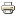# On the asymptotic uniqueness of bargaining equilibriaWorking paper
Author/s:
Arkadi Predtetchinski
Issue number:
RM/09/021
Publisher:
Maastricht University
Year:
2009
The paper studies the model of multilateral bargaining over the alternatives rep- resented by points in the m–dimensional Euclidean space. Proposers are chosen randomly and the acceptance of a proposal requires the unanimous approval of it by all the players. The focus of the paper is on the asymptotic behavior of subgame perfect equilibria in pure stationary strategies (called bargaining equilibria) as the breakdown probability tends to zero. Bargaining equilibria are said to be asymp- totically unique if the limit of a sequence of bargaining equilibria as the breakdown probability tends to zero is independent of the choice of the sequence and is uniquely determined by the primitives of the model. We show that the limit of any sequence of bargaining equilibria is a zero point of the so–called linearization correspondence. The asymptotic uniqueness of bargaining equilibria is then deduced in each of the following cases: (1) m = n− 1, where n is the number of players, (2) m = 1, and (3) in the case where the utility functions are quadratic, for each 1 ≤ m ≤ n−1. In each case the linearization correspondence is shown to have a unique zero. Result 1 has been established earlier in Miyakawa  and Laruelle and Valenciano . Result 2 is subsumed by the result in Predtetchinski . Result 3 is new.
Developed by Paolo Gittoi